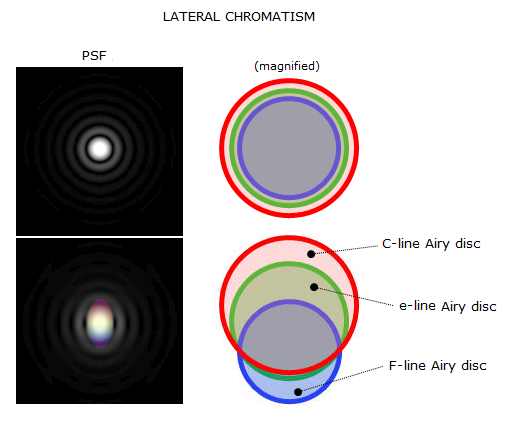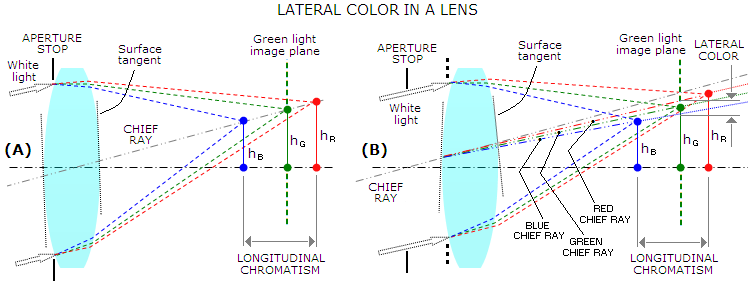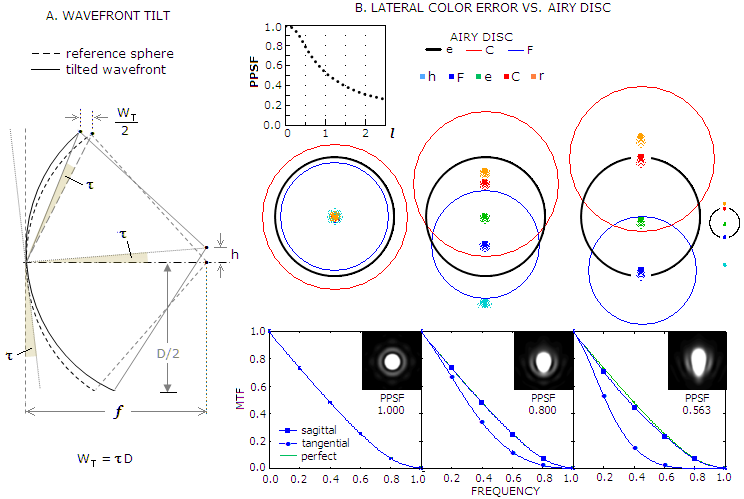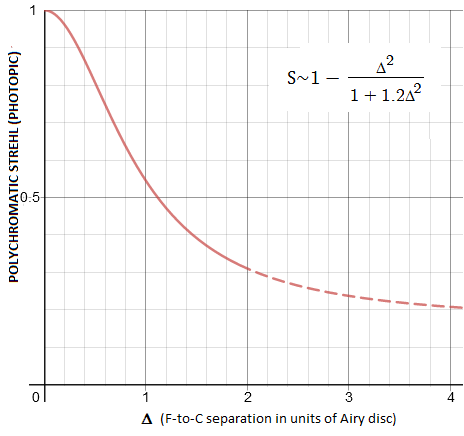telescopeѲptics.net          ▪▪▪▪                                             CONTENTS

# 4.7.2. Lateral color error

Origins of lateral color error - also known as transverse chromatic aberration - are not as obvious as for longitudinal chromatism. In simplest terms, it is the consequence of unequal refractive compensation at lens surfaces. Since different wavelengths refract at a different rate, a single refractive surface will always splitoblique incident light into a fan-like spread of wavelengths, diverging at slightly different angles. Unless this divergence is compensated for at another refracting surface, or surfaces, by the time this diverging light hits the image plane, the spread of wavelengths (i.e. colors) can be significant. It doesn't take much; for a f/10 lens, two wavelength will arrive at the image plane separated by the Airy disc diameter when their angle differential at the exit from the lens nearly equals the angular Airy disc diameter, or as little as 2.8 arc seconds.

Lateral color also can be generated by fabrication error, specifically, by wedge-like orientation of refractive surfaces relative to each other. Following text will limit to lateral color associated with oblique incidence pencils, but general principles are the same for both.

Since the reference ray for all aberrations resulting from obliquity of incident pencils is their chief ray, the chief rays of optimized wavelengths are unavoidably directed toward image plane at different angles (FIG. 71). For that reason, lateral color is sometimes referred to as chief ray chromatism.FIGURE 71: Cause of lateral color in a lens. With aperture stop at the lens (A), chief ray of the incident white light passes near the lens' center (not quite through the center, as usually depicted for thin lens and shown at left, but the difference is negligible with respect to lateral color). The white light chief ray splits into chief rays of different wavelength after the first surface, but the angle of divergence is very small, resulting in negligible height differential at the second surface. Due to surface tangents at the respective refraction points being nearly parallel, this section of lens acts as plano-parallel plate, with the slight differential in angular direction of color chief rays compensated for by their slightly different rate of refraction at the second surface. as a result, the chief rays travel toward focal plane at nearly identical angle, nearly equal to the incident angle, staying tightly together. Note that due to different focal lengths, chromatic difference of magnification for different colors is present in their respective focal planes. However, since all chief rays arrive at nearly identical angle, there is no lateral color in the green light focal plane: the other colors are merely defocused. When the aperture stop is displaced, either longitudinally or laterally, the geometry changes (B). The white chief ray is now directed farther off the lens center, with the tangents on two lens surfaces at  the respective points of refraction being no longer nearly parallel. As a result, refraction at the second surface is no longer compensatory, and the chief rays of different colors keep diverging toward focal plane. Consequently, they reach different heights in the green light focal plane, producing lateral color error. This error is now combined with longitudinal defocus, i.e. other colors are both, defocused and shifted laterally. Obviously, correcting longitudinal chromatism would only eliminate defocus error - as well as chromatic difference of magnification due to it - but wouldn't affect lateral color error, nor chromatic difference of magnification resulting from it.

As illustrated above, the two main determinants of lateral color error are stop position and lens shape. If, for instance, the 2nd surface tangent in (B) was nearly parallel to that at the 1st surface (a weak positive meniscus), the lens would have acted as plano parallel plate, producing negligible lateral color despite displaced stop.

Some basic relations for the above simple lens case help define specific factors of its lateral color. For the white light incidence angle α, the height at which it hits first surface is h1L, L being the longitudinal displacement of aperture stop. The angle of incidence at the first surface β=α1, with ρ1=h1/R1 being the angle formed by optical axis and the 1st surface's radius of curvature R1. The refracted angle, for small β, is β'=(n/n')β, with n, n' being the refractive index of incident and refractive medium, respectively. For lens in air, n=1 and β'=β/n'; since n' varies with the wavelength, so do their respective angles of refraction.

In other words, chief ray divergence that would produce lateral color is initiated at the first surface. In order to cancel this divergence, the tangent at refraction point on the second lens surface needs to be nearly parallel to that on the first surface, i.e. ρ12~0, with ρ1=h1/R1 and ρ2=h2/R2, where h2=h1+β't, t being the lens thickness (obviously, the curvature radii for first and second surface, R1 and R2, respectively, need to be of the same sign).

Consequently, in first approximation, the chief ray angle of refraction after the second surface is given by β"=n'(ρ12). In other words, for any non-zero value in brackets, the angle of divergence for any specific wavelengths will equal the product of that value and the glass refractive index n' corresponding to the wavelength. For any two wavelengths 1 and 2, the angle of their lateral divergence is:

δ1,2 = β"1-β"2 = (n'1-n'2)(ρ12)           (50)

For given aperture, focal length - or focal ratio - of the system is irrelevant to the magnitude of lateral color error, since the angular size of this error is constant (i.e. the linear extent of lateral color error remains constant with respect to the Airy disc size).

With multiple lens systems the calculation is more complex, but the principle remains the same: cancellation of lateral color requires the sum of refracted angles at its surfaces to be near zero for a given range of wavelengths.

As a wavefront aberration, lateral color error is a consequence of wavefront tilt vs. reference sphere. As monochromatic aberration, wavefront tilt does not affect point-image quality, only its location; however, in a wavefront that splits chromatically through refraction, tilt error varying with the wavelength does cause spread of energy in the central diffraction maxima (FIG. 72). Unlike secondary spectrum and spherochromatism, where most of the energy lost to the central maxima goes to the rings area, lateral color error mainly expands (and deforms) the central maxima.FIGURE 72
: (A) Simple geometry of wavefront tilt shows that the P-V error is given by W
T=τD, with the tilt angle τ =h/f, where h is the linear shift of point image in the image plane, f being the focal length (for object at infinity; image separation for close objects), and D the aperture diameter. The P-V to RMS wavefront error ratio is 432/3. The angle of tilt τ is determined by the angular discrepancy between chief ray angle for specified wavelength, and chief ray angle of the reference wavelength (in the visual context, usually around green e-line); it equals the angle of lateral divergence δ, as defined above, with the only difference being that it expresses the error relative to the primary wavelength. (B) The effect of lateral color error on point-image quality and overall contrast depends on its magnitude and spectral sensitivity of the detector. Shown is its effect on polychromatic PSF (PPSF, for 0.4-0.7μm range, photopic eye sensitivity) and MTF, in terms of C/F separation in units of e-line Airy disc diameter, Δ (on the graph marked as l). The system used for raytracing has negligible other aberrations (50mm f/9.56 Maksutov camera, R1=-206, S1=20.1, BK7, R2=217.5, S2=666, air, R3=-996, mirror, all mm, stop at the 1st surface), hence the effect is nearly entirely the result of lateral color error. The C/F separation needs to be half the e-line Airy disc radius, or less, for the polychromatic Strehl to remain within diffraction limited range. The primary effect on polychromatic PSF is elongation of the central maxima in the direction of lateral color shift, wider on the side of longer wavelengths' shift (top), narrower at the side of shorter wavelengths' shift (bottom). Asymmetrical expansion of the central maxima causes largest MTF contrast drop in the high-frequency range, from the maximum in the orientation coinciding with lateral shift (tangential) to near-zero in the orientation perpendicular to it (sagittal).

Tolerance for lateral color error, obviously, depends on the spectral sensitivity of detector. For photopic eye sensitivity, diffraction-limited maximum is at the C/F separation nearly equaling the e-line Airy disc radius; for even sensitivity over the visual range, polychromatic Strehl drops to 0.80 at only 30% of that separation.

As the PPSF/Δ graph on FIG. 72B indicates, the effect of lateral color error is not proportional to its angular magnitude. Similarly to secondary spectrum, negative effect of lateral color on image quality changes at a slower rate than its nominal magnitude. For instance, at C/F separation equaling half the Airy disc radius, the P-V wavefront error of primary spherical aberration corresponding to the resulting 0.81 Strehl is slightly better than 1/4 wave. Doubling the C/F separation does not double the corresponding P-V wavefront error, which is 1/2.4 waves P-V for 0.54 Strehl. Doubling it once more, to twice the Airy disc diameter, only lowers the polychromatic Strehl to 0.32, with the corresponding primary spherical aberration error of 1/1.8 wave P-V.A close empirical approximation for the photopic polychromatic Strehl resulting from the lateral color error is given by SP~1-Δ2/(1+1.2Δ2), with Δ being the C/F separation in units of e-line Airy disc diameter. The difference vs. raytracing values is within 1% of the nominal PPSF value for Δ<1, and doesn't exceed a few percentage points at Δ~2 (e.g. 0.31 vs. 0.32 by raytrace for Δ=2). The plot has quasi-Gaussian shape, with the drop in the Strehl becoming asymptotical for larger F-to-C separations - a consequence of the rate of wavelength divergence decreasing exponentially toward the central wavelength (the dashed portion of the plot indicates range where the approximation may not be accurate).

▐